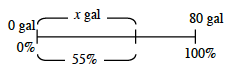### Home > MC2 > Chapter 4 > Lesson 4.1.1 > Problem4-10

4-10.

A fish tank that holds $80$ gallons of water is $55\%$ full. 4-10 HW eTool

1. Create a drawing like Louis’s in problem 4-4 to represent this situation.2. How many gallons are in the tank now? How many more gallons are needed to fill the tank?

Use equivalent ratios to solve this problem

$\frac{x}{55} = \frac{80}{100}$

Think about a Giant One that you could use to find the value of $x$.

To find how many gallons are needed to fill the tank subtract the number of gallons in the tank now from the total number of gallons the tank holds.